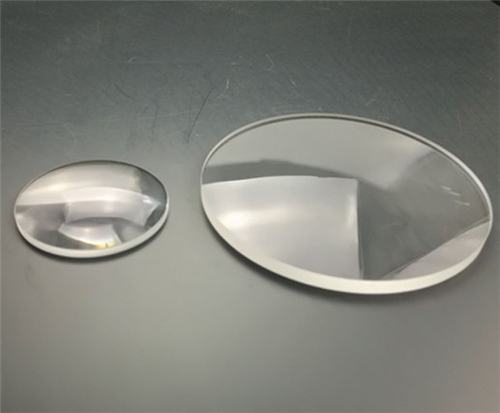# How to calculate the focal length of the lens?

Jan. 24, 2022

## Types of Lenses

There are two basic types of lenses: convex lenses and concave lenses. Convex lenses are thicker in the middle than at the edges and cause light to converge to a single point. Concave lenses are thicker at the edges than in the middle and cause light to diverge.

Convex and concave lenses have different configurations. A plano-convex lens is flat on one side and convex on the other, while a biconvex (also called a biconvex) lens is convex on both sides. A flat-concave lens is flat on one side and concave on the other, while a double-concave (or biconcave) lens is concave on both sides.

A combined concave-convex lens called a concave-convex lens is often referred to as a positive (converging) curved-moon lens. The lens is convex on one side and concave on the other, with the radius of the concave side being greater than the radius of the convex side.

A combination convex-convex lens called a convex-concave lens is usually called a negative (diverging) bent-moon lens. This type of lens has a concave and convex side just like a concave-convex lens, but the radius of the concave side is smaller than the radius of the convex side.## Focal length physics

The focal length f of a lens is the lens to the focal point F. Rays of light traveling parallel to the optical axis of a convex or concave-convex lens (of a single frequency) will meet at the focal point.

The convex lens converges the parallel rays to a focal point with a positive focal length. Because the light rays pass through the lens, the positive image distance (and the real image) is located on the side of the lens opposite the object. The image will be inverted relative to the actual image (upside down).

A concave lens diverges parallel light rays away from the focal point with a negative focal length, creating only a virtual smaller image. Negative image distance creates a virtual image on the same side of the lens as the object. The image will be oriented in the same direction as the original image (face up), only smaller.

## Focal length formula

To find the focal length using the focal length formula, one needs to know the distance u from the original object to the lens and the distance v from the lens to the image. the lens formula says that the reciprocal of the distance to the object plus the distance to the image equals the focal length f. This equation is written mathematically as

\frac{1}{u}+\frac{1}{v}=\frac{1}{f}

Sometimes the focal equation is written as

\frac{1}{o}+\frac{1}{i}=\frac{1}{f}

where o is the distance from the object to the lens, i is the distance from the lens to the image, and f is the focal length.

The distance is the distance from the object or image to the pole of the lens.

## Focal length example

To find the focal length of the lens, measure the distance and substitute the number into the focal length formula. Make sure the same measurement system is used for all measurements.

Example 1: The measured distance from the lens to the object is 20 cm, and the distance from the lens to the image is 5 cm. Completing the focal length formula yields.

\frac{1}{20}+\frac{1}{5}=\frac{1}{f} \\\text{or}\; \frac{1}{20}+\frac{4}{20}=\frac{5}{20} \\\\text{reduce the sum to get }\frac{5}{20}=\frac{1} {4}

Therefore the focal length is 4 cm.

Example 2: The measured distance from the lens to the object is 10 cm, and the distance from the lens to the image is 5 cm. The focal length equation shows that

\frac{1}{10}+\frac{1}{5}=\frac{1}{f} \\\text{then}\; \frac{1}{10}+\frac{2}{10}=\frac{3}{10}

Reducing this gives.

\frac{3}{10}=\frac{1}{3.33}

Thus, the focal length of the lens is 3.33 cm.